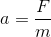# AP Physics B : Understanding Newton's Laws

## Example Questions

### Example Question #2 : Newton's Second Law

A potted plant is hanging from a rope attached to a hook. The plant exerts 25N of force. Assume the rope is weightless.

If the packaging says the rope is able to withstand 800N of force, what is the maximum amount of mass that can be hanging from the rope?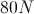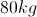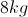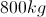Explanation:

If the rope can only withstand 800N of force, we can solve for the mass of the object which would result in this maximum force when hanging from the rope.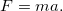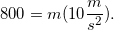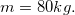### Example Question #3 : Newton's Second Law

A skydiver with a mass of 100kg jumps out of an airplane and reaches a terminal velocity of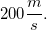.

Which of the following factors will not decrease the total force on the man as he jumps from the plane?

The man jumps as the plane is descending sharply

The man immediately opens his parachute

The man orients his stomach toward the ground, rather than his feet

Increasing the man's mass

Increasing the man's mass

Explanation:

The equation for force isThis shows that by increasing the man's mass, his force will increase after jumping from the plane.

All other options will result in a decreased acceleration when falling. Falling with his stomach downward increases the surface area of the diver, which increases his air resistance. A parachute will also increase the air resistance and surface area. If the plane is descending when he jumps, his time to reach terminal velocity will be reduced, as he will already have an initial velocity in the downward direction.

### Example Question #404 : Mcat Physical Sciences

A skydiver with a mass of 100kg jumps out of an airplane and reaches a terminal velocity of.

What is the total force acting on the skydiver at this point in his jump?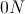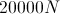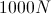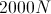Explanation:

The man has reached a terminal velocity, which means that he has an acceleration of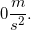Because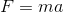, the total force on the skydiver is 0N. At this point, the force due to gravity in the downward direction is equal to the force of air resistance in the upward direction.

### Example Question #1 : Understanding Newton's Laws

A man pushes a crate across a frictionless floor at a constant velocity. Which of the following statements is true?

The force the man exerts on the crate is equal and in the same direction as the force the crate exerts on the man

The force the man exerts on the crate is equal and opposite to the force the crate exerts on the man

The force on the crate is greater than the force on the man

The force on the crate is less than the force on the man

The net force on the man is greater than zero

The force the man exerts on the crate is equal and opposite to the force the crate exerts on the man

Explanation:

According to Newton's third law, the force on the crate must have the same magnitude as the force on the man. The forces must also be in opposite directions. We can conclude that the net force on the man is zero, since he is moving with the crate at a constant velocity.

### Example Question #5 : Newton's Second Law

A 2000kg car with a velocity of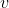collides head on with a 6000kg truck with a velocity of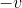. Which vehicle experiences the greater force? Which experiences the greater acceleration?

The truck experiences the greater force and the greater acceleration

The car and the truck experience equal force and the car experiences greater acceleration

Both the car and the truck experience equal force and acceleration

The truck experiences the greater force and the car experiences the greater acceleration

The car experiences the greater force and the greater acceleration